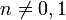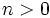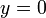# Bernoulli differential equation

## Definition

In normalized form, this first-order first-degree differential equation looks like:$y' + p(x)y = q(x)y^n$

where$n \ne 0,1$. (Note that the cases$n = 0,1$ give first-order linear differential equations).

### Solution method and formula

Divide both sides by$y^n$. If$n > 0$, this means that we may be potentially discarding the stationary solution$y = 0$, and must remember to add that back to the solution family at the end.

We get:$\frac{y'}{y^n} + \frac{p(x)}{y^{n-1}} = q(x)$

Now put$w = 1/y^{n-1}$ to get:$\frac{w'}{1 - n} + p(x)w = q(x)$

Multiply by$1 - n$ to get:$w' + (1 - n)p(x)w = (1 - n)q(x)$

This is now a first-order linear differential equation in$w$, and can be solved to get a family of functional solutions for$w$ in terms of$x$. Plugging back$w = 1/y^{n-1}$ gives a family of functional solutions for$y$ in terms of$x$. We can now add back$y = 0$.